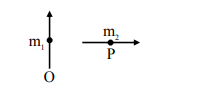# Two short magnetic dipolesQuestion:

Two short magnetic dipoles $\mathrm{m}_{1}$ and $\mathrm{m}_{2}$ each having magnetic moment of $1 \mathrm{Am}^{2}$ are placed at point $\mathrm{O}$ and $P$ respectively. The distance between OP is 1 meter. The torque experienced by the magnetic dipole $\mathrm{m}_{2}$ due to the presence of $\mathrm{m}_{1}$ is ...... $\times 10^{-7} \mathrm{Nm}$.Solution:$\vec{\tau}=\overrightarrow{\mathrm{M}}_{2} \times \overrightarrow{\mathrm{B}}_{1}$

$\tau=\mathrm{M}_{2} \mathrm{~B}_{1} \sin 90^{\circ}$

$=1 \times \frac{\mu_{0}}{4 \pi} \frac{M_{1}}{(1)^{3}} 1$

$=10^{-7} \mathrm{~N} . \mathrm{m}$

Ans. $1.00$